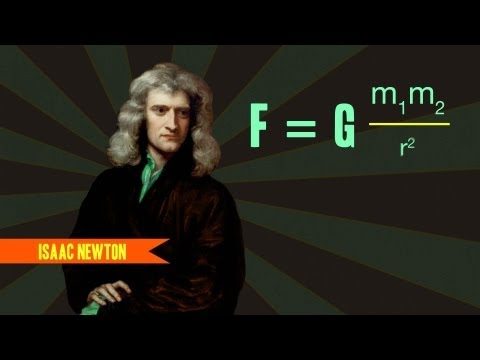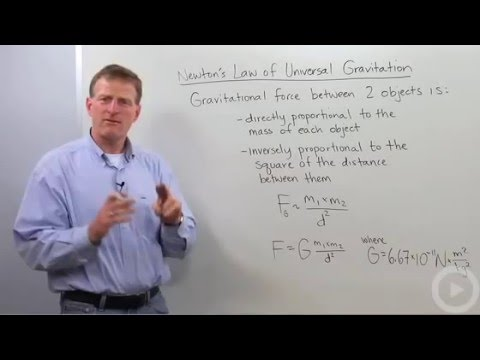## Gravitation

Subject: Science

#### Overview

The force of attraction between any two bodies in the universe is called gravitation. This note has information about universal law of gravitation, its effect and variables that affect gravitational force.

##### Gravitation

The force of attraction between any two bodies in the universe is called gravitation.

Force is that push or pull which changes or tends to change the state of rest or uniform motion in a straight line.

### Newton's law of gravitation

Newton's law of gravitation states that everybody in this universe attracts every other body with a force which is:

1. Directly proportional to the product of their masses i.e. F ∝ m1m2...(i) and
2. Inversely proportional to the square of distance between their centers i.e F ∝ 1/$d^2$ ..(ii)Combining Eqn (i) and (ii), we get

F ∝ $\frac{m1m2}{d^2}$

Or, F = ${G}\frac{m1m2}{d^2}$

Where G is the proportionality constant which is known as universal gravitational constant.

Numerically,

G = 6.67 X $10^{-11}$

$\therefore F\; = \; G \frac{m_1\times \;m_2}{d^2}$ .....(iii)

Universal gravitational constant G

The force of gravitation that is exerted between two bodies of unit mass placed at a unit distance from their centers.

i.e. m1 =1 kg

m2 = 1 kg

d = 1m

Then from Eqn (iii), we get

F=${G}\frac{1kg * 1kg}{1m^2}$

Or, F = G

G = F

Thus, the gravitational constant 'G' is numerically equal to the gravitational force 'F' when two masses of 1kg are separated by 1meter.

This law is called universal law because this law is applicable to all objects of any size (larger or small) all across the universe (terrestrial or celestial)

Value of G on other planets:

The value of G anywhere in the universe is 6.67 x 10-11. It is because G is universal constant and its value remains same everywhere in the universe.

Q: Two objects with a certain masses placed at a certain distance are ones separated by air medium and then by water medium. In which case is gravitational force greater:

A: Since the gravitation force depends upon mass and the distance between masses and does not depend on the medium where two objects are kept, gravitational force is equal in both air and water medium.

Effect on gravitational force of attraction if:

1. The masses of both objects is doubled keeping distance constant?

According to the question:

m1= 2m1

m2 = 2m2

D = d

∴ New force of attraction(fn)

fn =${G}\frac{2m1 * 2m2}{d^2}$  ...From Eqn iii

Or, fn = 4 ${G}\frac{m1 * m2}{d^2}$

Or, fn= 4F

Hence the new force will be 4 times more than the original force.

2. The masses of both objects is tripled keeping distance constant

According to the question:

m1 = 3m1

m2 = 3m2

D = d

∴ new force of attraction

fn = ${G}\frac{3m1 * 3m2}{d^2}$ ...From Eqn (iii)

fn = 9${G}\frac{m1 * m2}{d^2}$

Or, fn = 9F

Hence the new force will be 9 times more than the original force.
3. The mass of one body is doubled with distance reduced to half

According to the question:

m1 = 2m1

m2 = m2

D = d/2

∴ new force of attraction

fn = ${G}\frac{2m1 * m2}{(d/2)^2}$

Or, fn= ${G}\frac{2m1 * m2}{d^2/4}$

Or, fn = 2 ×4 ${G}\frac{m1 * m2}{d^2}$

Or, fn = 8F From Eqn (iii)

Hence the new force will be 8 times more than the original force

4. The mass of one body increased fourfold, that of other decreased by one-third with distance increased three times

Let,

Two masses are m1 and m2

Distance of separation is d

∴ The mutual force of attraction,

F = ${G}\frac{m1m2}{d^2}$........ (1)

According to the question:

m1 = 4m1

m2= 1/3 x m2

D = (3 x d)

∴ new force of attraction

fn = ${G}\frac{4m1*(1/3)m2}{(3d)^2}$ From Eqn (1)

Or, fn =4 ${G}\frac{m1*m2}{3*9d^2}$

Or, fn = $\frac{4}{27}$ ${G}\frac{m1m2}{d^2}$

Hence the new force will be 4/27 times less than the original force.

5. The masses of both is doubled and distance increased by double?

According to the question:

m1 = 2m1

m2 = 2m2

D = 2d

∴ new force of attraction

fn= ${G}\frac{2m1*2m2}{(2d)^2}$ From Eqn (1)

Or, fn =${G}\frac{m1*m2}{d^2}$

Or, fn = F from Eqn (1)

Hence the new force will be equal to the original force.

Factors Affecting Gravitational force:

The factors affecting gravitational force are as follows:

Since,

F = ${G}\frac{M1M2}{d^2}$ therefore, the affecting factors are:-

Mass of the two bodies' i.e. F∝M1M2 when mass increases gravitational force increases.The distance between their centers

i.e. $F\propto\frac{1}{d^2}$when the distance increases gravitational force decreases.

##### Things to remember
• The force of attraction between any two bodies in the universe is called gravitation.
• Newton’s law of gravitation is F=G.$\frac{M_1M_2}{d^2}$
• Universal gravitation constant G law is applicable to all objects of any size (larger or small) all across the universe (terrestrial or celestial)  F=G.$\frac{M_1M_2}{d^2}$
• It includes every relationship which established among the people.
• There can be more than one community in a society. Community smaller than society.
• It is a network of social relationships which cannot see or touched.
• common interests and common objectives are not necessary for society.
##### Videos for Gravitation##### Gravitation: The Four Fundamental Forces of Physics #3##### Newton's Law of Universal Gravitation##### Newtons Universal Law of Gravitation - Science in a Minute
The gravitational force (F) produced between two heavenly bodies is directly proportional tothe product of masses of the two heavenly bodies (m1 and m2) and inversely proportional to the square of the distance (d) between them.
i.e.Gravitational constant is the force of gravitation between two bodies each with a mass of 1kg placed at a distance of 1m apart.

Here,
Mass of one body (m1)= 10 kg
Mass of second body (m2) = 20 kg
Distance between them (d) = 10 meter
Gravitational force (F)= ?
We have,or,or,or, F = 6.67 × 2 × 10-11
or, F = 1.334 × 10-10 N
Hence, the gravitational force between two masses of 10 kg and 20kg if they are at 10m distance is 1.334 × 10-10 N.

Here,
Mass of the sun (m1) = 2 × 1030kg
Mass of the earth (m2) = 6 × 1024 kg
Distance between the centres of the earth and the sun (d) =1.5 × 1011 m
Force of attraction (F)= ?
According to the Newton"s law of Gravitation,= 6.67 × 10-11 ×= 3.56 × 10 22 N
Hence, the force of attraction between the sun and the earth is 3.56 × 10 22 N.

Given,
Mass of the earth (M)= 6 × 1024 kg
Radius of the earth (R) = 6400 km
= 64 × 105 m
= 6.4 × 106 m
Weight of the man (F)= 977 N
Mass of the man (m)= ?
Universal gravitational constant (G) = 6.67 × 10-11 Nm2/kg2
According to the formula,or,===999.951012-13
=99.99kg.
Hence, the mass of the man is 99.99kg.

Here,
Gravitational force of attraction between two bodies is given by:When the mass of each body is doubled then new masses will be m1 = 2m1 and m2 = 2m2 and distance is tripled, then d = 3dor,or,or,The magnitude of the force reducesof the initial force.

Newton"s law of gravitation states that "The force of attraction gravitation between any two bodies in the universe is directly proportional to the product of their masses and inversely proportional to the square of the distance between their centers".
Solution:
Given,
Distance between the moon and the earth (d) = 3 × 105 km
= 3 × 108 m
Mass of the moon (M) = 7 × 1022 kg
Pulling force of the moon (F) = ?
According to the formula,[G = 6.67 × 10-11 Nm2/kg2]

==== 5.18 × 105 N

Every kilogram of water of river is attracted by 5.18 × 105N of force of the Moon.

Let,
Mass of the first object = m1
Mass of second object = m2
Distance between two objects = d
Force between two objects= F
We have,……….(i)
Here,
The distance between two objects is doubled,
So, d = 2d
Now,or,The magnitude of force reduce toof the initial force i.e. force is decreased by 4 times when distance between the objects is doubled.
If the mass of two objects of 1 kg each is kept at a distance of 1m, the value of gravitational force (F) will be 6.67 × 10-11 N.
By calculation,
Mass of the first object (m1) = 1kg
Mass of second object (m2)= 1 kg
Distance between two objects (d) = 1 m
Gravitational force (F) = ?
We have,or,(where, G = 6.67 × 10-11Nm2/kg2)
=6.67 × 10-11N
F =6.67 × 10-11 N.
The force of attraction between the bodies and the earth in which earth pulls the bodies towards it's center is called gravity

We can say that Free fall is the cause and weightlessness is an effect. Similarly Free fall is the condition of body falling freely towards the earth's surface without any external resistance but weightlessness is the condition of having the weight of a body zero.During freefall the weight of the body is zero.

The force of gravity acts on the centre of the earth or any other objects whose gravity acts on other smaller object.